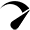# Learning Derivative and its Daily Life Applications

## Derivative

Contents

In mathematical terms, differentiation is defined as the method of finding the derivative of a function. While in physics terms it is stated as the process in which the rate of change in a quantity is calculated with respect to the other one.

Differentiation finds the instant rate of change in one variable based on changes in another. For example, velocity is the change in the rate of displacement with time as the prime component. However, this change in speed is not the estimated average at each instant.

This can be said as the same as slope, i.e. the rate of change of distance over time. In a similar manner, the differentiation defines several physical quantities such as max and min values of a function, acceleration and the tangent along the curve.

## Mathematical Notation

Mathematically we can define the differentiation process as the dy/dx, expressing the change in variable y with respect to x as the other variable. Therefore, if y = f(x) then the f’(x) is given as the dy/dx. Whereas, the derivative of the function f(x) is given as the f’(x)

### Differential Calculus

Differentiation of a function is an essential branch of calculus and forms the sub-branch namely differential calculus. Where differential calculus is the study of the instant rate of changes within a function by incorporating the concepts of limits and derivatives.

Try the dy/dx calculator with steps to get the differential value of the implicit function without passing through the complex calculations.

### Directional Calculus

The directional derivative is the essential branch of differentiation. It is the instantaneous rate of change of a function at a given point along with its direction. It is one of the complex but amazing concepts of derivatives. Such types of directional derivatives can calculate by using the formula of directional derivatives or by using the directional derivative calculator.

### How to Learn Derivative

Learning differential calculus is crucial in solving problems pertaining to the derivative of a function, and must be comprehended well by mathematics students.

The extent to which it is necessary to learn differentiation to the same extent is easy to understand. The simplest way to learn differentiation is simply to understand its basic rules.

Simply, learn each and every differentiation rule one by one. However, the differentiation rules are just representations of more basic notions such as derivative of a function’s sum, power and product.

Using the differentiation rules therefore you better grasp the differential calculus concepts. Learning the basic differentiating concepts and using them to practice sums would let you imply them in daily life problems as well.

Putting these key concepts into practice can assist you in finding solutions to problems. The basic differentiation rules that form the fundamental concepts of differential calculus includes

• ### Sum Rule

If y = u(x) ± v(x), then dy/dx = du/dx ± dv/dx

• ### Product Rule

If y = u(x) × v(x), then dy/dx = u.dv/dx + v.du/dx

• ### Quotient Rule

If y = u(x) ÷ v(x), then dy/dx = (v.du/dx- u.dv/dx)/ v2

• ### Chain Rule

Let y = f(u) be a function of u and if u=g(x) so that y = f(g(x)

Then d/dx(f(g(x))= f'(g(x))g'(x)

• ### Constant Rule

y = k f(x), k ≠ 0, then d/dx(k(f(x)) = k d/dx f(x)

## Daily Life Applications of Derivative

The process of differentiation is not upto theoretical concepts of calculus rather its notions are significantly applying in real life as well. Several fields of study including, engineering, mechanics and various physical and social sciences constitute the differential rules.

By determining the rate of change concept, finding the tangent and normal curve equations and finding the largest and smallest value of functions on a graph the process of differentiation can applied to various real-life problems.

Calculus is the language and tool of economists and businessmen. Differentiation is using in economic research to examine functional relationships. Derivatives, or the change in one variable relative to another, are the same as economic marginalism.

Practically, in business and economics the differential concepts using graphs assist to determine the profit and loss. Calculus can calculate the total interest paid throughout the life of a loan.

The method of generating derivatives allows economists to assess the average change in income per year of increased education or experience. Derivatives also help managers optimize profitability and evaluate profit growth.

### Temperature Changes

In order to determine the temperature variations the notions of differentiation are typically utilizing. As the derivatives identify the rate of change over time, implying the same equations will use to determine the changes in temperature.

### Biology

In biology, derivatives may use to measure muscle contraction, medication absorption, and bacterial growth. By taking the derivative of the equation, biologists may determine the rate at which the virus is spreading. Differential equations are also helpful to link medication concentrations over time.

### Physical Quantities

The differentiation is the rate of change in a quantity with respect to another quantity. Therefore, to calculate the speed or distance travelled, such as miles per hour, kilometres per hour, i.e. magnitude of one variable depending upon the derivatives have used. Similarly, in physics, derivatives are utilizing to derive a large number of equations and quantities like velocity, displacement and volumes.

### Seismology

In the field of seismology, it is common practice to determine the magnitude range of an earthquake using differential equations.

error: Content is protected !!Optimized by Seraphinite Accelerator
Turns on site high speed to be attractive for people and search engines.